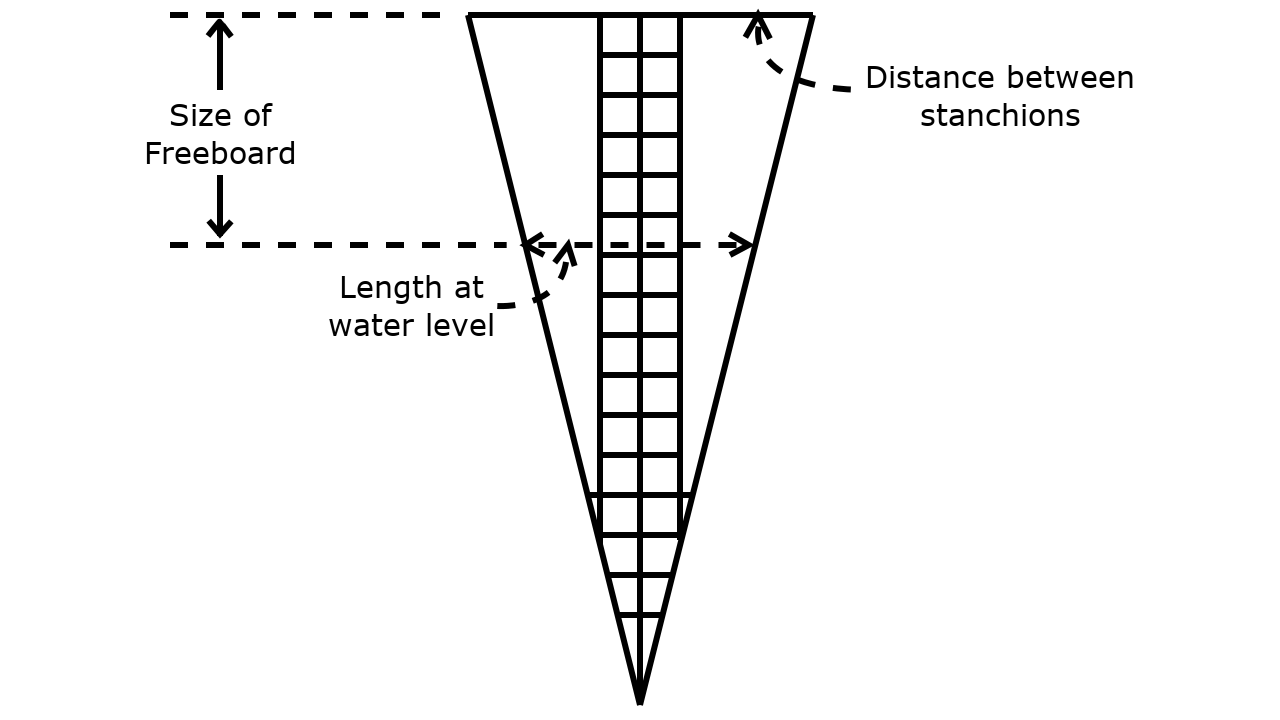# Sling DesignAs I am writing this, we have not tested this design, but it should give you ideas about making your own design

## Materials

• 30m of tape with a good breaking strain, please check this measurement using the calculations below
• UV resistant cotton
• UV resistant material
• 3 Rings - Used at the points of the triangle
• Rope - Used to secure the sling to the boat

## Measurements

The measurements that you will need are

• Distance between the stanchions - These stanchions need to be close to the mast and are where you will be rolling the casualty aboard
• Size of the freeboard - With this measurement make sure you are measuring the size of the freeboard, between the stanchions where you will be rolling the casualty aboard
• Length required at the water

With Salty Lass the distance between the stanchions was 1.8m (A) and the size of the freeboard was 1.2m (B). Now I decided that the length I wanted at the water was 1.2m (C), I chose this figure because I wanted a length that was sufficient to support the casualty and have their head supported to some extent. I then used these distances in the following equation

## D = (A * B)/(A - C)

This gave me the length of my design between the base of the triangle and the apex of the triangle (D), the sides (E) can be calculated using

## E = √((A/2)2+D2)

Now I had a width of 50cm (F) for my ladder and a step size of 25cm (G) that's the distance between the rungs. For the overlap I had a rather excessive overlap of 12cm (H) but I thought that is would be better to be safe than sorry plus for my own convenience I used 25cm (I) which is 2 x H + 1cm extra in the calculations below.

So the total length of tape required is

• 1 * (A + I) Base of sling
• 2 * (E + I) Sides of sling
• 3 * (D + I) Ladder sides
• (D/G) * (F + I) Ladder rungs

The fabric section that you require is

• D * (A - F)

This will give you too much fabric, but that is not a bad thing. I used cargo netting for this section but maybe a different solution would work here just as well.

## Hints and tips

These are the hints and tips that I have on making the sling

• Have somewhere flat that you can lay your sling out, this is so that you can make sure that all the straps are flat
• I used cargo net for my sides but maybe a different fabric could be used here
• I used a long zigzag stitch to secure the straps together, this worked really well as all I had to do was stitch forwards and then stitch backwards
• On the sections that I wanted to intersect I would measure out the space that I wanted and put a pin at the end of the pin. I would then repeat the process on the other strap. These two pins would then form two of the sides of a square for where the straps needed to be joined
• Only ever use new strapping as it makes it so much easier to sew
• Use needles that are designed for sewing Jeans together for this project
• One subscriber suggested having two rings at each point of the triagle, so the load at these points was shared

If I have any complains with my design then maybe another cross piece where the triangle section is would be a good thing